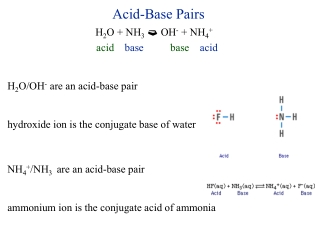DownloadDownload PresentationAcid-Base Pairs

# Acid-Base Pairs

Télécharger la présentation## Acid-Base Pairs

- - - - - - - - - - - - - - - - - - - - - - - - - - - E N D - - - - - - - - - - - - - - - - - - - - - - - - - - -
##### Presentation Transcript

1. Acid-Base Pairs • H2O + NH3 OH- + NH4+ • acidbase base acid • H2O/OH- are an acid-base pair • hydroxide ion is the conjugate base of water • NH4+/NH3 are an acid-base pair • ammonium ion is the conjugate acid of ammonia

2. HClO 4 + H2O  H3O+ + ClO4- • acid: HClO4 • conjugate base: ClO4- • HClO4/ClO4- are an acid-base pair • base: H2O • conjugate acid: H3O+ • H3O+/H2O are an acid-base pair • _________________________________________________________ • H2O + H2O  H3O+ + OH- • acid: H2O • conjugate base: OH- • H2O/OH- are an acid-base pair • base: H2O • conjugate acid: H3O+ • H3O+/H2O are an acid-base pair

3. conjugate acid-base pairs • acid: HCl • conjugate base of HCl: Cl- • acid: H2SO4 • conjugate base of H2SO4: HSO4- • acid: NH3 • conjugate base of NH3: NH2- • ____________________________________________________________________________________ • base: NH3 • conjugate acid of NH3: NH4+ • base SO42- • conjugate acid of SO42-: HSO4-

4. The stronger the acid, the weaker is it’s conjugate base • The weaker the acid, the stronger is it’s conjugate base • The stronger the base, the weaker is it’s conjugate acid • The weaker the base, the stronger is it’s conjugate acid • Strong acid HI > HBr > HCl >> HF Weak acid • Strong Base F- >> Cl- > Br - > I- Weak Base • Acid strength depends on bond strength & stability of the resulting anion: HF H+ + F - • Table 10-2

5. Ternary Acids • Ternary Acid - An acid that contains hydrogen, oxygen and a non-metal • most ternary acids are hydroxyl compounds of nonmetals (oxoacids): HNO3 can also be written as HONO2 • H2SO4 can also be written as (OH)2SO2 .. .. O || N .. .. O O H .. .. .. hydroxyl groups hydroxyl group

6. Acid Strength of Ternary Acids • HNO3 H+ + NO3- • Why does nitric acid lose a proton instead of a hydroxide ion? • The E.N. = 0.5 (N & O) and 1.4 (O & H) • Why is HNO3 a strong acid and HNO2 (HONO) a weak acid? • look at the ox. # Nitric Acid N +5 ox. state Nitrous Acid N +3 ox. state .. .. 3.0 O || N 3.0 .. N 3.5 3.5 2.1 .. 2.1 .. .. O O H O .. O .. .. H .. .. .. hydroxyl group hydroxyl group

7. Acid Stregth of HClOx series x= 1, 2, 3 or 4 • hypochlorous, chlorous, chloric and perchloric acid • HClO HClO2 HClO3 HClO4 • +1 +3 +5 +7 • HClO4 >> HClO3 > HClO2 > HClO • What does this tell us about their conjugate bases? • ClO4- << ClO3- < ClO2- < ClO -

8. Make a hypothesis on the acid strength of HBrO3 compared to HIO3 • Br and I are in the same oxidation state (+5) • The ternary acid with the most electronegative non-metal • will be the most acidic • HBrO3 > HIO3

9. Acidic Salts & Basic Salts • Normal Salt - A salt that doesn’t have ionizable hydrogens or hydroxyl groups. • Acidic Salt - A salt that has ionizable hydrogens but, doesn’t necesarily produce acidic solutions. • H2CO3 + NaOH  NaHCO3 + H2O • H2CO3 + OH- HCO3- + H2O • Polyprotic Acid rxn w/ < stoich. amount of SB gives an acidic salt

10. Basic Salt - A salt that has basic hydroxyl groups but doesn’t necesarily create basic solutions. • Fe(OH)2(s) + HCl  Fe(OH)Cl(s) + H2O • Fe(OH)2(s) + H+ + Cl- Fe(OH)Cl(s) + H2O • polyhydroxy base rxn w/ < stoich. amount of SA gives a basic salt

11. Preparation of Acids • Binary Acids - hydrogen halides • nonvolatile + salt of  salt of + volatile acid volatile acid nonvolatile acid acid • H2SO4 + NaF(s) NaHSO4 + HF(g) • H3PO4 + NaBr(s) NaH2PO4 + HBr(g) • Ternary Acids • nonmetal oxide + water  ternary acid • N2O5(s) + H2O  2HNO3 • Binary & Ternary Acids • PX3 + 3H2O  H3PO3 + 3HX • PX5 + 4H2O  H3PO4 + 5HX